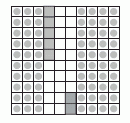Email us to get an instant 20% discount on highly effective K-12 Math & English kwizNET Programs!

#### Online Quiz (WorksheetABCD)

Questions Per Quiz = 2 4 6 8 10

### Grade 4 - Mathematics9.4 Decimal Problems

 Converting decimals to fractions Write the number without the decimal point. Divide the number with as many tens as the number of digits after the decimal point. Example: Convert 0.75 to a fraction. Numerator - 75 Denominator - 100 (since there are 2 numbers after the decimal point) Answer: 75/100 Adding decimals Add 0.35 and 0.42Take a square divide into hundred parts. Mark 0.35 by shading 3 tenths and colouring 5 hundreths. Similarly mark o.42 by shading 4 tenths and colouring 2 hundredths. Now count the total number of tenths and hundredths in the square. Answer: 0.77 Subtracting decimalsWrite the decimals one below the other as shown. Subtract as shown. Answer: 1.76 Directions: Answer the following questions. Also write at least 5 examples of your own for each of the following: * converting fraction to decimal * adding two decimals * subtracting two decimals
 Q 1: What number would be next? 2.3, 2.4, 2.5 . . . .2.6262.83 Q 2: Which is the correct way to write five and eight hundredths?8.055.085805.8 Q 3: Fill in the blank 400.99 - 399.09 =1.81.9399.08 Q 4: Which number is greater 15.2 or 15.0215.0215.2 Q 5: What is the sum of .39 + .67?1061.02.961.06 Q 6: Subtract the decimals. 34.95 - 27.99 =6.965.966.69 Q 7: What decimal is between 12.4 and 12.6?12.112.312.512.7 Q 8: Which is the correct way to write one and two tenths?1.22.12.011.02 Question 9: This question is available to subscribers only! Question 10: This question is available to subscribers only!